Design: propeller turbine, screw, aerial, marine, turbine, tidal, wind, kaplan, foil, wings, 3D. Discover heliciel software: Modeling turbine kaplan in heliciel guide vanes draft tube turbine
hydropower turbine propeller or Kaplan 1/3: Description and operation of a turbine system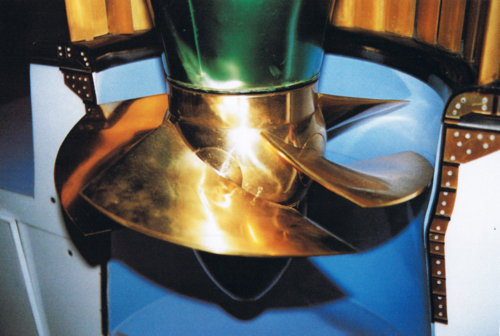Chapter Summary hydroelectric turbines::

Introduction:
The capture of hydropower is an example of inventiveness and creativity, developed by man to mate with his world. Principles and physical phenomena, tamed through the centuries, have given rise to today's most modern power systems. In the race for clean energy, the creation of a hydraulic turbine is a means of access to energy that can be located and dimensioned in perfect harmony with its natural site. But the profitability of the project requires a method of rational design of the hydraulic system organs. Many small sites powers are neglected because the turbine design uses methods engineering calculations that may discourage contractors. Although the literature is rich in this area, it is reserved to a mathematician public. But mathematics is a reflection inspired by natural phenomena, it must therefore be possible to describe in natural language.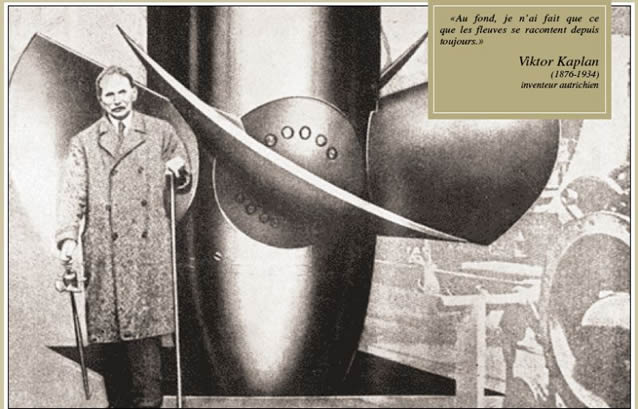The calculations are essential for system design seriously, so we use two economic software tools, mecaflux and Heliciel that support the purely mathematical part.We can stay focused on an overview of the physical phenomena, producing energy transformations. This article gives a complete methodology and design tools, whose goal is to bring within the reach of everyone, the design of a turbine and the various organs of a mini hydropower plant.

turbine kaplan in heliciel

• Provide an accessible method, mainly based on the theory of propeller design. This methodical approach is original, because using the theory of propellers, it can sizing all organs for optimum performance, according to the specifications.
• Propose economic software tools for all calculations and implementation of 3D shapes of the blades of the turbine, without handling Complex equations: propeller design (Heliciel) and calculation of head losses (mecaflux)
• To design control organs of an efficient turbine through a tutorial example..
This Article remains open and will enhance with happiness of your contribution(_contact@heliciel.com_)
The mathematical foundations necessary for understanding the rest were grouped on a page that can be accessed in parallel as a reminder: Quick reminder on the relationship Power, Energy, Work, Flow.

Sommaire:
1. Part 1: Parameters and operating parts of a turbine system.
2. Part 2: The relation stator guide vanes and propeller
3. Part 3: The design method of hydraulic turbine capture
4. Part 4: Tutorial designing a turbine system.
5.

1: Part1 Parameters and organs for operating a turbine system.
Types of turbines:Capture the energy of the water takes various forms, to mate with the available power of the site. The key parameters of the hydroelectric plant being the height of fall and the flow rate, there are 2 types of turbines, which differ according to these parameters::
Impulse turbines Impulse turbines that operate solely with the kinetic energy of the fluid(speed energy) at ambient pressure, such as a Pelton turbine, adapted to great heights falls, and low flow, or turbineCROSSFLOW: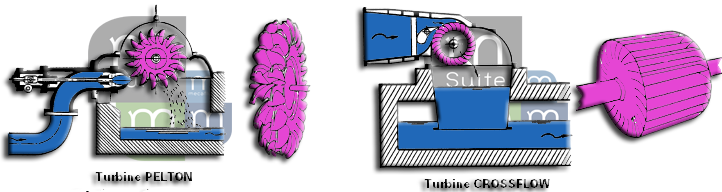Reaction turbines combining the kinetic energy (speed) and the pressure energy (pressure difference between the inlet and the outlet of a turbine in a fluid pipe):Francis turbine is suitable for heights and medium speeds, Kaplan turbine, or propeller is suitable for low head and high flow rates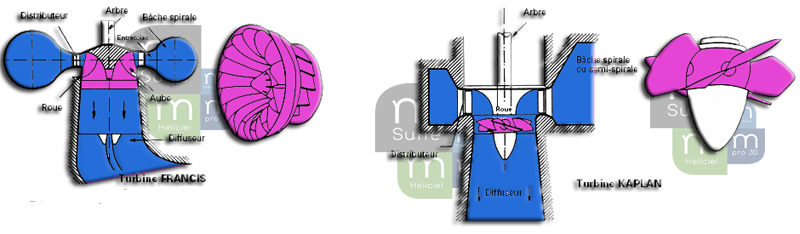Heliciel The site is dedicated to the design of propellers, so only to turbine design type bulbs, Straflo, propeller or Kaplan, using propellers turbines to turn the hydraulic power into mechanical power, will be detailed.
The hydraulic power of the site and the power generated:

Ph hydraulic power in watts, of the site depends:

• The difference in height of fall H in meters, between the surfaces of the upstream and downstream basin.
• The flow rate Q in m3/sec, through the system.
P(raw)= Q(m/s) x H(m) X ρ(kg/m3) x g(m/sec²)

with :

ρ density en kg/m3
g 9.81 en m/s2
Q turbine flow in m3/s
Let us explain by the concept of gross head and net head:
During its displacement from the upstream basin to the downstream basin, the fluid will undergo friction, and encounter obstacles that will reduce its energy. This energy loss in pipes is called head loss and is expressed in meters ... The head loss represents the energy lost in the pipes. This loss reduces the hydraulic power as if the height of drop was lower:So we distinguish the net power and raw power according to the height of drop(net or gross) included in the calculation formula of the site power:P(w)= Q(m/s) x H(m) X ρ(kg/m3) x g(m/sec²)

The relation speed, pressure, altitude:
the axial velocity of the fluid (parallel to the axis of rotation) in our turbine speed would be calculated according to the relation: V(m/sec)=Q(m3/sec) / S(m²) with:
• V: velocity of the fluid passing through the propeller(m/sec)
• S:Section swept by the propeller(m²)
• Q: volume flow rate (m3/sec)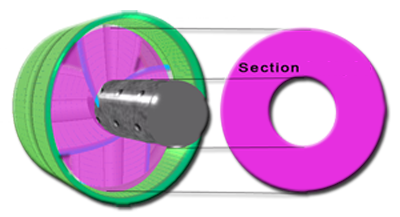In passing through the turbine, the fluid will transmit energy to the shaft through the negative pressure generated by the blades. The energy lost by the fluid, resulting in a loss of speed and pressure between the upstream and downstream of the propeller. A given operating flow rate will require a pressure upstream of the propeller greater than the pressure absorbed by the propeller. This requires that the net head in the flow rate of operation is sufficient to compensate the delta pressure generated by the turbine. (we will ignore for the moment the effect of the draft tube or the diffuser)

How much power will we get ? To obtain electric power, we must first change our hydraulic power into mechanical power to the propeller shaft, and then into electrical power, using a generator. Organs performing these transformations being not perfect, it will cause energy losses.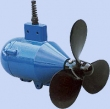• The passage of hydraulic energy, mechanical energy, determines the organs of our hydropower plant. We detail below, the design of these organs. The turbine efficiency is the ratio between the hydraulic energy captured, and converted mechanical energy.

• The generator converts mechanical energy into electric energy . The generator efficiency is the ratio of the mechanical energy of the shaft and the electrical energy produced.
• generator efficiency = Electrical power delivered(w) / shaft power(w)

• The overall system efficiency is the ratio between the hydraulic energy of the site, and the electrical energy supplied..
• overall system efficiency =Electrical power delivered(w) / hydraulic power(w)

Quick overview of three main organs of the hydraulic system:.
So we distinguish three main organs: "turbine guide vanes, draft tube" embodying the process of hydraulic energy into mechanical energy:
1: The propeller or turbine: This is the rotating part on the shaft, which converts fluid energy into mechanical energy..The propeller, owing to the shape of its blades, transforms the kinetic energy (speed energy) of the fluid stream into mechanical energy. If the propeller captured all the kinetic energy of the fluid, the fluid would not have velocity when leaving the turbine. This would imply that, deprived of all movements fluid would accumulate and clog the outlet of the turbine. So we must leave a little bit of kinetik power to allow the fluid circulation. This energy allowed to fluid, thus limiting the performance to a maximum : the Betz limit (see theory froude capture energy), ie a maximum yield of 0.6. This means that our propeller will, in the best case, get that 0.6 times the energy of the fluid,and this only if the orientation of its blades is an optimum angle of incidence with the fluid..

Yet we speak of hydraulic turbines offering performance of around 0.8 see 0.9, so why this difference? The fact that the fluid is in pipe gives us the ability to control the speed and direction of pressure fluid through the organs recover some of the energy that can not capture the turbine in open water, about 0.4 times the total energy of the fluid. We must distinguish the efficiency of the propeller alone (0.6 max), from the efficiency of the overall system "turbine guide vanes, draft tube" which can reach 0.9.

2:turbine guide vanes or stator: This is a crown of airfoils mobile, which direct fluid and control its rotation (the tangential component of the fluid velocity)according to its degree of openness. The guide vane or stator can also be used as flow control valve..
Guide vanes stator turbine type bulb: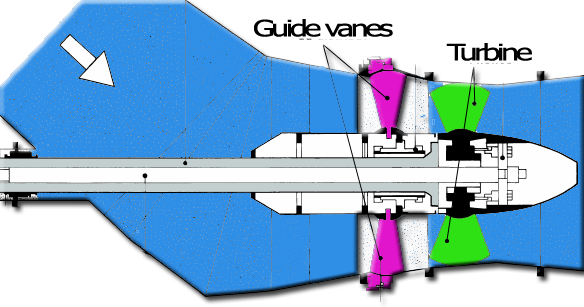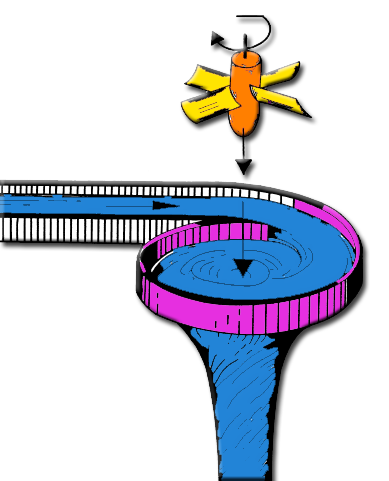The Kaplan turbine guide vanes, is generally placed at the center of volute favoring the formation of the vortex and ensuring uniform distribution of flow rate around the guide vanes.

The fins of the ring-type guide vanes (bulbs) totally ensure the rotation of the flow while the guide vanes, integrated into a volute, complement the work of the volute which already sets the flow rotation. The deviation caused by blades is a source of pressure drop it must be minimum. A strong deviation implies the choice of a the volute-type guide vanes

3: the draft tube: This divergent cone generates suction using the residual velocity, at the turbine outlet . This recovery system of kinetic energy is detailed in the chapter "Designing the draft tube"After this brief presentation of the main organs of a hydroelectric turbine, see how to size these organs and evaluate the performance of our site: calculation method

Chapter Summary hydroelectric turbines::